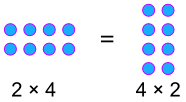Definition of

# Commutative LawThe Law that says you can swap numbers around and still get the same answer when you add.
Or when you multiply.

Examples:
You can swap when you add: 6 + 3 = 3 + 6
You can swap when you multiply: 2 × 4 = 4 × 2